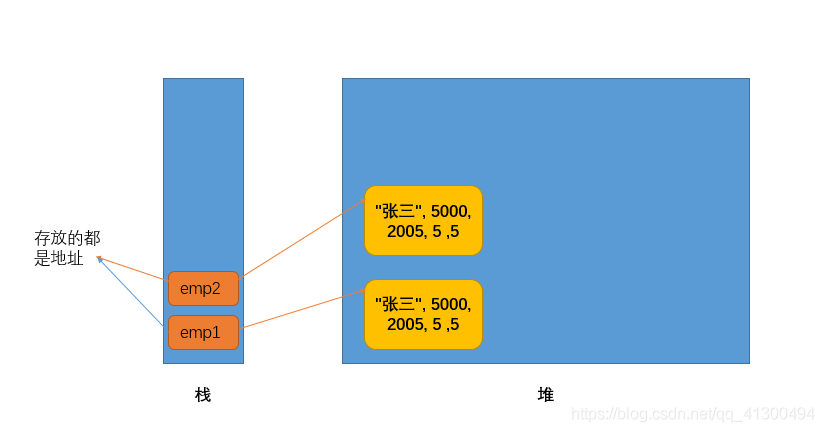# The differences between the equals method in the string class and the equals method in the object class

#### 1. First, let’s look at the equals method

in Object class

``````public boolean equals(Object obj) {
return (this == obj);
}
``````

we can see if the Object class is judging the same Object, let’s take a look at the following example

``````Employee emp1 = new Employee("张三", 5000, 2005, 5 ,5);
Employee emp2 = new Employee("张三", 5000, 2005, 5 ,5);
System.out.println(emp2.equals(emp1));
``````

stop here and think about what’s going to come out?

, I’m sure a lot of beginners like me think it will output true. But the answer is false. Why?We’re looking at the only line of code in the body of the method

``````        return (this == obj);
``````

, what does this==obj stand for?In fact, what we’re doing here is determining whether this is the same address as obj, or whether it’s the same object in the heap.it is easy to see that emp1 and emp2 do not store the same address in the stack, so it will print false.

2. Next, let’s look at the equals method in the String class

``````public boolean equals(Object anObject) {
//判断是否同一个对象
if (this == anObject) {
return true;
}
//判断anObject 是否是String的实例对象
//如果是的话判断两个String对象的长度是否相等
//如果相等再判断每一个字符是否相等
if (anObject instanceof String) {
String anotherString = (String)anObject;
int n = value.length;
if (n == anotherString.value.length) {
char v1[] = value;
char v2[] = anotherString.value;
int i = 0;
while (n-- != 0) {
if (v1[i] != v2[i])
return false;
i++;
}
return true;
}
}
return false;
}
``````

The equals method in the

String class does two things. The first method is the same as the equals method in Object. If not the same object, then determine whether the length is equal, then determine whether each character is equal.